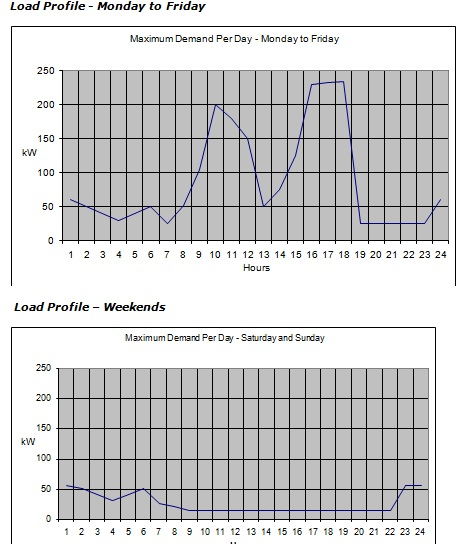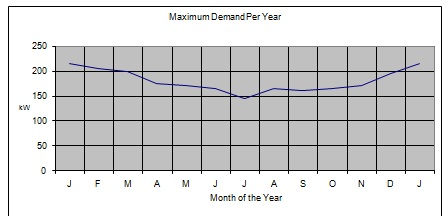+61-413 786 465

info@mywordsolution.com

## Engineering

 Civil Engineering Chemical Engineering Electrical & Electronics Mechanical Engineering Computer Engineering Engineering Mathematics MATLAB Other Engineering Digital Electronics Biochemical & Biotechnology

problem 1: Describe the construction of different kinds of power transformer?

describe with the help of diagrams the given in relation to the construction of power transformers. Include the merits and demerits in your explanation.

a) Core type.
b) Shell type.
c) Sandwich wound.
d) Concentric wound.

problem 2: Recognize the operating principles of a power transformer under no-load and load conditions: A 230/1000V 50 Hz, single phase transformer has the given test data:

Open circuit test (L.V.)
Primary voltage = 230V
Primary current = 1.30 A
Input power = 115W
Short circuit test (H.V.)
Primary voltage = 50 V
Secondary current = 5.55A
Input power = 165 W

a) Find out and sketch the equivalent circuit for the transformer?

b) Find out the efficiency of the transformer at full load current at a power factor of unity and 0.8 lagging?

c) Find out the percentage regulation at a power factor of unity and 0.8 lagging?

problem 3: Discuss the modes of connection for polyphase transformers?

Discuss with help of electrical grouping and wiring connection diagrams the various kinds of connections for polyphase transformers listed below:

a) Dd 6
b) Dz 0
c) Yd 1
d) Yz 11

problem 4: Discuss the factors governing the tariff structures?

Describe three various industrial tariff structures; include details of the type of business which would suit each tariff structure.

problem 5: Analyze the methods for reducing energy consumption? Find out the cost of energy used in a system?

The energy consumption for a small factory is given at the end of the brief.

a) Three commercial tariffs from an energy supplier are shown below. Using these (or other commercially available tariffs), determine the most suitable tariff for the factory detailed. Your answer must be supported by mathematical analysis.

Tariff A:
Standing charge per quarter of £21.95 and every unit is charged at 19.93 ppu
Maximum demand is charged at £6.95 per KVA per annum

Tariff B:
All units are charged at 25.70 ppu
Maximum demand is charged at £5.95 per KVA per annum

Tariff C:
First 1500 units in a quarter is charged at 21.82 ppu
All additional units in the quarter charged at 18.49 ppu
Maximum demand is charged at £7.45 per KVA per annum

b) Propose changes to the working practices of the factory detailed in order to reduce the energy consumption and the cost of the energy bill.

Factory Details:

The factory is fed and metered at 400/230V three-phase and neutral. It comprises of a main factory area, a storage warehouse for incoming raw materials and final products awaiting delivery and a small office block. The major intake position is located at one end of the factory and sub-main feeds are taken to the warehouse and office block.

The factory is on one level with big open doors at one end where goods and materials are moved to and from the warehouse. This is constructed of brick walls with a high single pitched roof and few windows.

The warehouse is a tall building of similar construction to the factory with two roller shutter doors, one for deliveries of raw materials and one for distribution of the finished product.

The office block is a brick building with a flat timber and felt roof. This has recently been refurbished. New double glazed windows were fitted and the electrical supplies and the lighting scheme were brought up to modern standards.

1 x three-phase 45kW electrode boiler for heating a large volume of water to a temperature of 95oC for product washing; the water is then flushed down to the sewerage system

1 x three-phase 22kW processing unit operating with a total power factor of 0.71 lagging.

7 x three-phase 9kW pump motors operating at 0.82 power factor lagging.

12 x single-phase 2.25kW motors, various uses, operating at 0.78 power factor lagging.

16 x single-phase 3kW wall mounted forced convector heaters operating at 0.92 power factor lagging.

9kW of fluorescent discharge lighting operating at 0.76 power factor lagging employing magnetic choke switch start circuits fitted with standard discharge tubes.

Warehouse:

5kW of high bay high pressure mercury vapor lighting operating at 0.75 power factor lagging.

7 x 3kW block storage heaters.

Office:

8kW of single-phase power feeding computer equipment and vending machines.
3 x 2.25kW storage heaters
2.5kW of high frequency electronic ballast fluorescent lighting operating at 0.97 power factor lagging.

Energy Consumption Profile:

The three graphs shown point out the load pattern for the whole factory complex. The load profile for Monday to Friday and Saturday and Sunday can be supposed to be reasonably constant all through the year. Seasonal changes can be recognized by using the third graph as this shows the loading based on a weekly maximum demand.This can be taken that the initial cost of static, loss-free capacitors, comprising that of the essential control gear is £65.00 per kVAr. The annual interest and depreciation charges total 12% per annum.

The total number of units consumed throughout the 12 month period 2011 was 384,125.

Electrical & Electronics, Engineering

• Category:- Electrical & Electronics
• Reference No.:- M9450

Have any Question?

## Related Questions in Electrical & Electronics

### Problem 1given a sequence xn for 0lenle3 where x0 1 x1 1

Problem # 1: Given a sequence x(n) for 0≤n≤3, where x(0) = 1, x(1) = 1, x(2) = -1, and x(3) = 0, compute its DFT X(k). (Use DFT formula, don't use MATLAB function) Problem # 2: Use inverse DFT and apply it on the Fourier ...

### Case studythis assignment consists of a written report of

CASE STUDY This assignment consists of a written report of approximately 1000 words and any diagrams in which you are asked to critically compare different process methods used to achieve the same result and show an awar ...

### Questions -q1 a single-phase transformer rated 21 kv130 v

Questions - Q1. A single-phase transformer rated 2.1 kV/130 V, 7.8 kVA has the following winding parameters: r1= 0.7Ω, x1 = 0.9Ω, r2 = 0.04Ω and x2 = 0.05Ω. Determine: a. The combined winding resistance ________ Ω and le ...

### 1 a name the three major groups of contamination and

1. (a) Name the three major groups of contamination and briefly describe their physical characteristics. (b) Where do the above contamination types come from? Give one example of each. 2. Name two processes metrics which ...

### Summative assessmentin 2017 sej101 assessment will consist

Summative Assessment In 2017 SEJ101 assessment will consist of nine tasks that will develop a portfolio of your assessed work. Throughout the trimester you will have the opportunity for feedback on all nine tasks before ...

### Questions -problem 1 - determine the laplace transform ofa

Questions - Problem 1 - Determine the Laplace transform of: (a) cos(ωt + θ) (b) sin(ωt + θ) Problem 2 - Obtain the Laplace transform of each of the following functions: (a) e -2t cos(3t)u(t) (b) e -2t sin(4t)u(t) (c) e - ...

### Problems -problem 1 - find v0 in the op amp circuit of fig

Problems - Problem 1 - Find v 0 in the op amp circuit of Fig. 1. Problem 2 - Compute i 0 (t) in the op amp circuit in Fig. 2 if v s = 4 cos(10 4 t). Problem 3 - If the input impedance is defined as Z in = v s /I s , find ...

### Nanotechnology engineering - resonance circuits questions

Nanotechnology Engineering - Resonance Circuits Questions - Q1) A series RLC network has R = 2KΩ, L = 40mH and C = 1μF. Calculate the impedance at resonance and at one-fourth, one-half, twice, and four times the resonant ...

### Question 1for the ce amplifier in figure 1 given the

Question 1 For the CE amplifier in Figure (1), given the following component parameters: Parameter Value β DC , β AC 150 V BE 0 . 7 V V CC 12 V R C 820 ? R E 1 100 ? R E 2 220 ? R 1 20 k? R 2 5 . 2 k? R L 100 k? C 1 , C ...

### Question 1 a pnp transistor withnbspbeta 60 is connected

Question 1. A pnp transistor with β = 60 is connected in a common-base configuration as shown in figure P5.8 (a) The emitter is driven by a constant-current source with I E = 0.75 mA. Determine I B , I C , α, and V C . ( ...

• 13,132 Experts

## Looking for Assignment Help?

Start excelling in your Courses, Get help with Assignment

Write us your full requirement for evaluation and you will receive response within 20 minutes turnaround time.

### Why might a bank avoid the use of interest rate swaps even

Why might a bank avoid the use of interest rate swaps, even when the institution is exposed to significant interest rate

### Describe the difference between zero coupon bonds and

Describe the difference between zero coupon bonds and coupon bonds. Under what conditions will a coupon bond sell at a p

### Compute the present value of an annuity of 880 per year

Compute the present value of an annuity of \$ 880 per year for 16 years, given a discount rate of 6 percent per annum. As

### Compute the present value of an 1150 payment made in ten

Compute the present value of an \$1,150 payment made in ten years when the discount rate is 12 percent. (Do not round int

### Compute the present value of an annuity of 699 per year

Compute the present value of an annuity of \$ 699 per year for 19 years, given a discount rate of 6 percent per annum. As